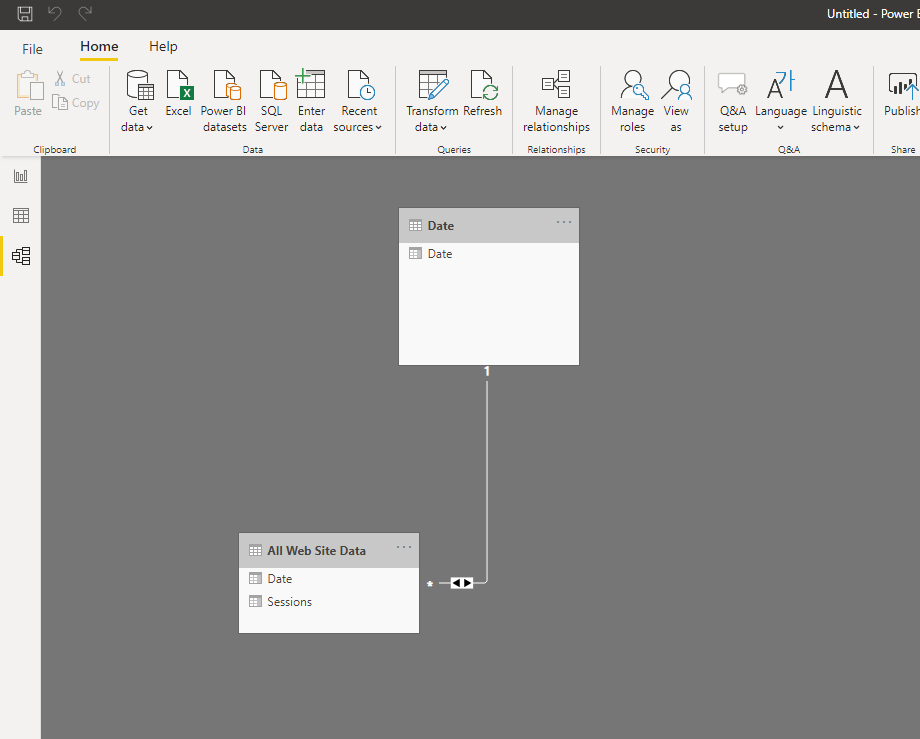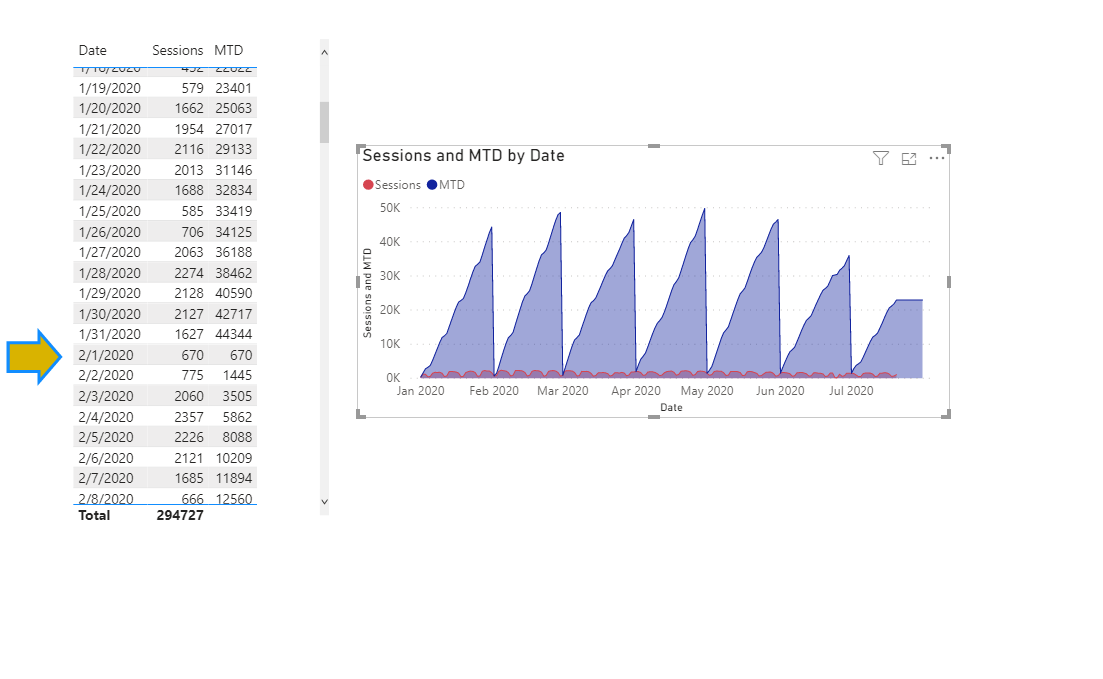# How to Calcuate MTD, QTD and YTD in Power BI

Calculating the month to date, year to date, or quarter to date is not as daunting as you think in DAX. However, there are few stepst that are needed before you can get these function to provide the information that you are looking for.  These are all a part of Time Intelligence Function in DAX.

What do you need?

1. Calendar Table
2.  Time Series Data

You might be wondering on why you need a Calendar Table. The reason that this is so important is that Time Intelligence function work off a continuous date field. So if your data has whole in it, the funcitons will not work. To give you an indication of this, I you didnt have a sale on 1/1/2020 and there is no date. This would create a whole in your data.

If you dont have a Calendar Table you can easily create one with the following Table function. You are going to go to New Table in the Modeling heading at top ribbon.

The CALENDAR function works like this CALENDAR(Start Date,End Date)

Date = CALENDAR(DATE(2020,1,1),DATE(,2020,12,31)

How to Set up Our Data Model

Now we can connect our date table to our fact table. Simplly click the relation icon and the far right and setup a 1 to many relation from the Date Tabel to the Fact Table.Use Time Intelligence Functions.

The functions that will be using are bellow:

1. SUM
2. CALCULATE
3. DATESYTD
4. DATESQTD
5. DATESMTD

The SUM function is the function I am using for this example however you can use any aggrgation you like. We will be using CALCULATE function to change the  context of the data. See the CALCULATE tutorial for more info.

## How to CALCULTE Month to DATE

To calculate Month to Date sessions for the website data in this example. Here is the syntax below:

CALCULATE(SUM(Sessions),DATESMTD(Date[Date])

In this able we are provide calcuate with aggrated column which is session and then we are using the DATESMTD function for our filter. The DATESMTD requires the Date column.

What does this do, it sums all the session up for the month and starts again at the next month.### Calcuate Quarter to Date and Year to Date

Use the same syntax above to create a YTD and QTD formula

CALCULATE(SUM(Sessions),DATESYTD(Date[Date])

CALCULATE(SUM(Sessions),DATESQTD(Date[Date])

For more detal watch the Video

• in
• |
• July 20, 2020#### Gaelim Holland

Subscribe
Notify of